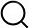# Also in the Article

Variable set size

Constructing graphs from genetic encodings
Sci Rep, Jun 24, 2021;

Procedure

In this section we expand the simplifying assumption of mapping networks with constant set sizes, allowing now for sizes to be distributed according to q(s). The method in the previous section, where we mapped with fixed set sizes, is a special case of this with $q(s)=δ(s-s0)$.

Given an edge between two sets of sizes $si$ and $sj$ drawn from q(s), $si∗sj$ edges are introduced into the node-level network. The expected number of links at the node level is therefore the number of rules multiplied by a factor of $⟨s1s2⟩=⟨s⟩2$. The expected density can then be expressed as

when $Nset⟨s⟩>>1$. Thus, the overall density is not expected to differ from the ER network, even with variable set sizes.

To derive the degree distribution, we consider a set with degree $kj$ at the set-level network. Such a set contains $sj$ nodes, each with the same degree $Kj=∑i=1ksi$. Depending on q(s) and the degree distribution $pk$ of the set-level network, the resulting expected degree distribution of the node-level network $pK$ is given by

According to the law of total expectation and the law of total variance,

and

If the set-level model is an ER network, having Poisson degree distribution with $σ2(k)=⟨k⟩$, (12) simplifies to $σ2(K)=⟨k⟩⟨s2⟩=⟨K⟩⟨s2⟩⟨s⟩$. The GM(ER) model under variable set size therefore has a degree distribution with Fano-factor (as a measure of dispersion) $σ2(K)⟨K⟩=⟨s2⟩⟨s⟩$.

Q&A